# ISEE Lower Level Math : How to find a proportion

## Example Questions

← Previous 1

### Example Question #1 : How To Find A Proportion

A cookie recipe that bakes 12 cookies calls for 3 eggs. How many eggs would be needed to make 36 cookies?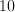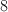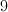Explanation:

36 cookies is 3 times more than 12. Multiply the number of eggs by 3 to get 9.

### Example Question #2 : How To Find A Proportion

Give the value ofthat makes this proportion statement correct: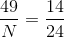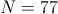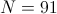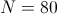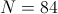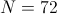Explanation:

Cross-multiply, then solve for: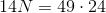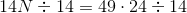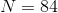### Example Question #3 : How To Find A Proportion

Give the value ofthat makes this proportion statement correct: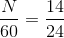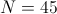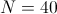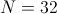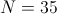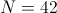Explanation:

Solve forby multiplying both sides by 60: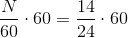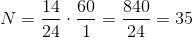### Example Question #4 : How To Find A Proportion

If 1 inch on a map represents 5 miles, how far apart, in miles, are two cities that are 6.6 inches apart on the map?

33 miles

24 miles

12 miles

36 miles

6.6 miles

33 miles

Explanation:

Set up a proportion with the inches on the map and physical miles: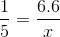Solve for x: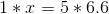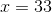### Example Question #5 : How To Find A Proportion

A group of 6th grade students at Manor Intermediate are going on a field trip to the local theme park.

There are 2 chaperones required for every 9 students on the field trip.  How many chaperones will need to attend the field trip if there are 27 students going?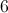chaperoneschaperoneschaperoneschaperoneschaperones

Explanation:

The best way to solve this problem is to set it up as two ratios that are equivalent to each other.  This forms a proportion.  Make sure to set up the ratios so that the relationships go together across the equal sign.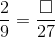Use cross products to then solve for the missing value.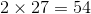Therefore, mental math or division can be used to find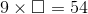.

Since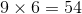,

there would need to be 6 chaperones attending the field trip.

### Example Question #6 : How To Find A Proportion

A bag of marbles contains 4 green marbles, 7 black marbles, and 5 red marbles. What is the proportion of green marbles?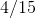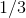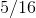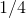Explanation:

First, sum the total number of marbles: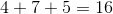Next, divide the total number of green marbles by the total number of marbles and simplify: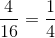### Example Question #7 : How To Find A Proportion

A bag has 11 green chips, 7 blue chips, and 6 red chips. What is the proportion of green chips?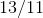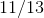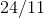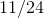Explanation:

The proportion is determined by dividing the number of selected items by the total number of items.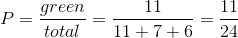### Example Question #8 : How To Find A Proportion

A drawer contains 5 pairs of blue socks, 2 pairs of white socks, 4 pairs of brown socks, and 7 pairs of black socks. What is the proportion of brown socks?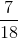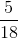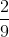Explanation:

A proportion is determined by dividing the number of a specific event by the total number of events: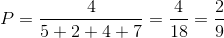### Example Question #9 : How To Find A Proportion

If Eric can read a page from his text book inminutes, how many pages can he read in 15 minutes?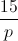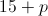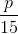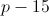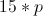Explanation:

The problem can be represented by the proportion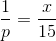.

Solve: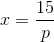Eric can read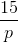pages in 15 minutes.

### Example Question #10 : How To Find A Proportion

If Kristin makes $300 in a week when she works 15 hours, how much would she make if she worked 24 hours next week at the same rate? Possible Answers: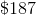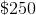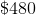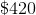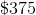Correct answer:Explanation: To find the rate at which she is currently paid, divide$300 by the hours she works, 15, to see that she earns $20 per hour. To find her earnings for next week, multiply 24 hours by$20 per hour to see that she will make \$480 next week.

Or set up the proportion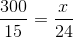and solve for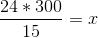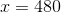← Previous 1